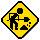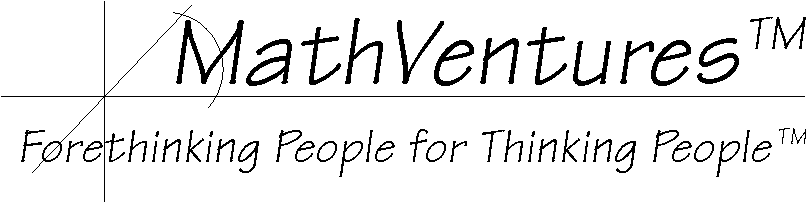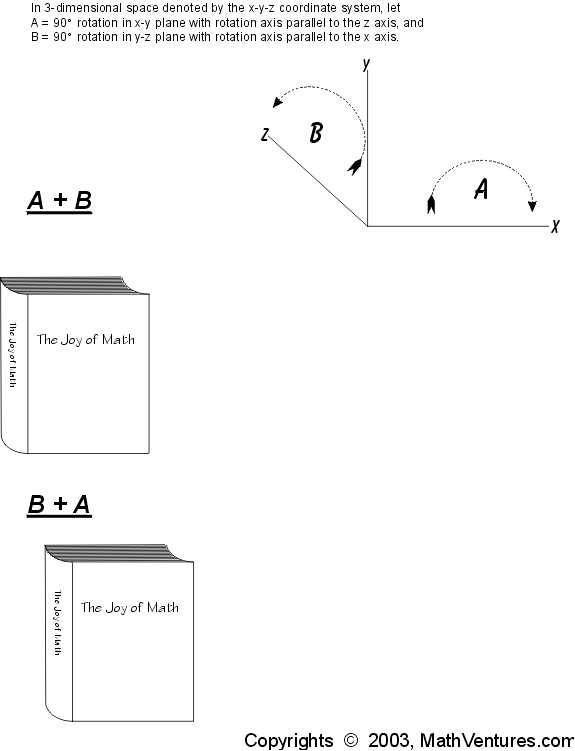Hard hat area, construction in progress# Logic  the Art of Reasoning

Mathematics   the Art of Studying Patterns Using Logic

# Here Is an Exception#### In other word, is

a + b = b + a

#### always true?

Here is an exception:
How about the graphic example below. In it, a and b are not simple numbers. Each of them is a 90 degree rotation and the addition operation is the result of two such rotations, the second of which must not be in the same plane as the first.#### As you see, in the graphic example above, the result of adding up these two 90 degree turns (rotations) is not the same. In other words,

a + b != b + a.
(if you are not familiar with th symbol  !=, it means not equal.)